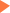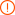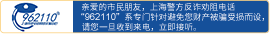# GRE数学冷门题型解题思路实例讲解

2019年07月30日15:51 来源：小站整理## GRE备考资料免费领取 请选择资料！

GRE数学考试中题型众多，除了大家比较熟悉的标准5选1单选题、图形题和大小比较题外，其实还有直接求解题、不定项选择题和错误判断题等考生可能比较陌生的题型。这些出现频率较低的题型考生假如不熟悉出题形式和方法也很容易出错。下面就由小编来逐一点评这些冷门题型的解题思路。

1. 直接求解题

The average(arithmetic- mean)of the 11 numbers in a list is 14. If the average of 9 of the numbers in the list is 9，what is the average of the other 2 numbers?

11个数的算术平均数是14。若其中9个数的算术平均数为9，则剩下的2个数的平均数是多少?

2. 不定项选择题

In triangle ABC，the measure of angle A is 25 and the measure of angle B is greater than 90. Which of the following could be the measure of angle C?

Indicate all possible values.

A. 12 B. 15C. 45 　D. 50 E.70

3. 错误判断题

The symbol ? represents one of the four operations- of addition, subtractio，multiplica-tion and division，and 3 ? 1 = 3.

For each of the following equations，indicate whether the equation must be true，must be false，or could be either true or false.

Equation

6 ? 2 = 3

6 ? 2 = 4

6 ? 2 = 12

Must Be True

Must Be False

Could Be True or False

GRE中出现的非常规数学符号可以看成ETS自己定义的一种运算。本题中，符号“?”代表的是加减乘除四种四则运算中的某一种，而且3?1=3，由这些已知条件可以推出?可以是乘，也可以是除。接下来就考察考生对于must be true、could be true这类逻辑判断语言的理解了。Must be true意为必然正确;Could be true意为可以正确，即存在正确的可能。

### 相关文章未输入验证码未输入验证码未输入验证码

## GRE30天热搜

GRE关键词沪公网安备 31010602002658号